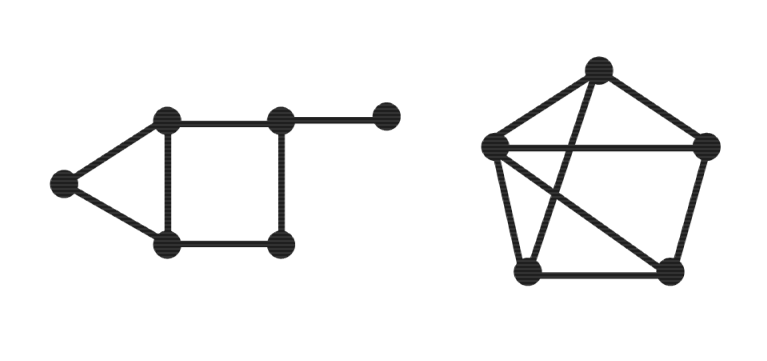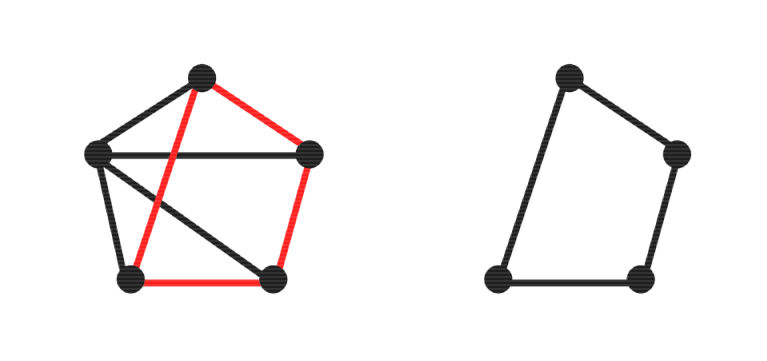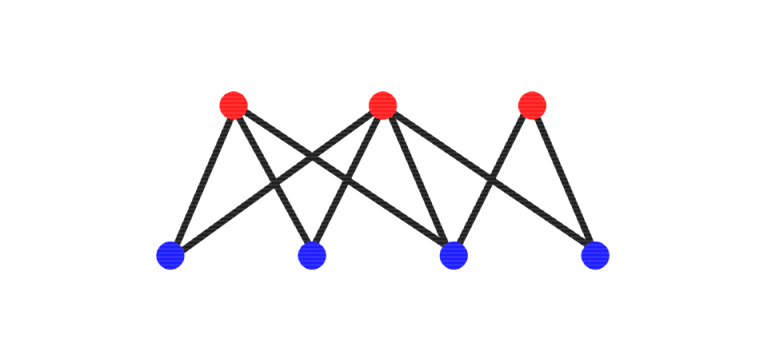# A combinatorial algorithm for finding maximum cuts

 Page 1 / 3
This report summarizes work done as part of the Wavelet Based Image Analysis PFUG under Rice University's VIGRE program. VIGRE is a program of Vertically Integrated Grants for Research and Education in the Mathematical Sciences under the direction of the National Science Foundation. A PFUG is a group of Postdocs, Faculty, Undergraduates and Graduate students formed round the study of a common problem. This module provides mathematical background on the maxcut problem and develops an exact branch and bound algorithm for the maximum cut of unweighted graphs that is designed for improved performance on sparse graphs.

## Introduction

Finding the maximum cut of a graph is a difficult to compute problem in combinatorial optimization with several applications in the world of engineering and physics. This research develops and evaluates an exact branch and bound algorithm for the maximum cut of unweighted graphs that is designed for improved performance on sparse graphs.

The module provides a general overview of the problem along with necessary mathematical background in "The Maxcut Problem" and a brief note on various approaches to the problem in "Several Algorithms" . "A New Algorithm" describes a new algorithm for finding maximum cuts. Results of empirical performance evaluation appear in "Empirical Testing" , which "Conclusion" further discusses.

## The maxcut problem

Before discussing the maxcut problem, it is necessary to provide some background information regarding relevant concepts in graph theory, the most fundamental of which is the graph itself. A graph $G\left(V,E\right)$ is an ordered pair comprised of a set of vertices V and a set of edges E that connect pairs of distinct vertices in V . Two examples are shown in [link] . Graphs may be either weighted, in which a real value is assigned to each edge, or unweighted, in which all edges have equal value. Although the former is more broadly applicable, further discussion will focus almost exclusively on the latter.Two example graphs appear above.

Unsurprisingly, a subgraph ${G}_{1}\left({V}_{1},{E}_{1}\right)$ of graph $G\left(V,E\right)$ is a graph with vertex set ${V}_{1}\subseteq V$ and edge set ${E}_{1}\subseteq E$ . Of particular usefulness will be the subgraph of $G\left(V,E\right)$ induced by a given set of edges ${E}_{1}\subseteq E$ , known as edge induced subgraph, which consists of that given set of edges E 1 along with all vertices ${V}_{1}=\left\{v|\left(u,v\right)\in ,{E}_{1},,,u,\in ,V\right\}$ that occur as an endpoint of at least one edge in E 1 . An example of an edge induced subgraph is shown in [link] .The subgraph induced by the red colored edges is shown on the right.

One class of graphs that will be especially important to discussion of the maxcut problem is bipartite graphs. A graph $G\left(V,E\right)$ is bipartite, like the example in [link] , if there are sets ${V}_{1},{V}_{2}\subseteq V$ such that ${V}_{1}\cup {V}_{2}=V$ , ${V}_{1}\cap {V}_{2}=\varnothing$ , and $\left(u,v\right)\in E$ only if $u\in {V}_{1},v\in {V}_{2}$ or $v\in {V}_{1},u\in {V}_{2}$ . Additionally, a graph is bipartite if and only if it has no subgraph that is a cycle of odd length.In the above bipartite graph, the vertices are colored red or blue to highlight the vertex partitions.

A cut of a graph can be informally understood and visualized as a closed curve crossing some realization of the graph where each edge can be crossed at most once, as seen in [link] . Notice that the curve partitions the graph vertices into two disjoint subsets located to each side of the curve.

#### Questions & Answers

What fields keep nano created devices from performing or assimulating ? Magnetic fields ? Are do they assimilate ?
Stoney Reply
why we need to study biomolecules, molecular biology in nanotechnology?
Adin Reply
?
Kyle
yes I'm doing my masters in nanotechnology, we are being studying all these domains as well..
Adin
why?
Adin
what school?
Kyle
biomolecules are e building blocks of every organics and inorganic materials.
Joe
anyone know any internet site where one can find nanotechnology papers?
Damian Reply
research.net
kanaga
sciencedirect big data base
Ernesto
Introduction about quantum dots in nanotechnology
Praveena Reply
what does nano mean?
Anassong Reply
nano basically means 10^(-9). nanometer is a unit to measure length.
Bharti
do you think it's worthwhile in the long term to study the effects and possibilities of nanotechnology on viral treatment?
Damian Reply
absolutely yes
Daniel
how to know photocatalytic properties of tio2 nanoparticles...what to do now
Akash Reply
it is a goid question and i want to know the answer as well
Maciej
characteristics of micro business
Abigail
for teaching engĺish at school how nano technology help us
Anassong
Do somebody tell me a best nano engineering book for beginners?
s. Reply
there is no specific books for beginners but there is book called principle of nanotechnology
NANO
what is fullerene does it is used to make bukky balls
Devang Reply
are you nano engineer ?
s.
fullerene is a bucky ball aka Carbon 60 molecule. It was name by the architect Fuller. He design the geodesic dome. it resembles a soccer ball.
Tarell
what is the actual application of fullerenes nowadays?
Damian
That is a great question Damian. best way to answer that question is to Google it. there are hundreds of applications for buck minister fullerenes, from medical to aerospace. you can also find plenty of research papers that will give you great detail on the potential applications of fullerenes.
Tarell
what is the Synthesis, properties,and applications of carbon nano chemistry
Abhijith Reply
Mostly, they use nano carbon for electronics and for materials to be strengthened.
Virgil
is Bucky paper clear?
CYNTHIA
carbon nanotubes has various application in fuel cells membrane, current research on cancer drug,and in electronics MEMS and NEMS etc
NANO
so some one know about replacing silicon atom with phosphorous in semiconductors device?
s. Reply
Yeah, it is a pain to say the least. You basically have to heat the substarte up to around 1000 degrees celcius then pass phosphene gas over top of it, which is explosive and toxic by the way, under very low pressure.
Harper
Do you know which machine is used to that process?
s.
how to fabricate graphene ink ?
SUYASH Reply
for screen printed electrodes ?
SUYASH
What is lattice structure?
s. Reply
of graphene you mean?
Ebrahim
or in general
Ebrahim
in general
s.
Graphene has a hexagonal structure
tahir
On having this app for quite a bit time, Haven't realised there's a chat room in it.
Cied
what is biological synthesis of nanoparticles
Sanket Reply
what's the easiest and fastest way to the synthesize AgNP?
Damian Reply
China
Cied
how did you get the value of 2000N.What calculations are needed to arrive at it
Smarajit Reply
Privacy Information Security Software Version 1.1a
Good
Got questions? Join the online conversation and get instant answers!
Jobilize.com Reply

### Read also:

#### Get the best Algebra and trigonometry course in your pocket!

Source:  OpenStax, The art of the pfug. OpenStax CNX. Jun 05, 2013 Download for free at http://cnx.org/content/col10523/1.34
Google Play and the Google Play logo are trademarks of Google Inc.

Notification Switch

Would you like to follow the 'The art of the pfug' conversation and receive update notifications?ByBy